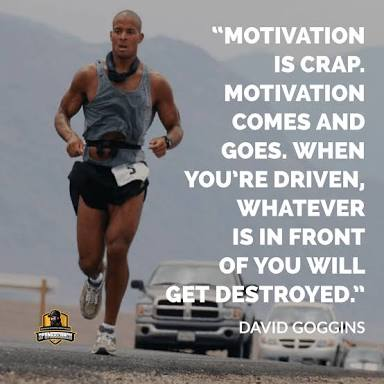# 9+ HOURS WORK/STUDY CHALLENGE (only for courageous titans)

Sharing code - tn1ii4
Current streak - 22 days
Highest streak - 112 days
Age - 20
Gender - M
Location - INDIA

# WELCOME WARRIORS.

This Challenge is only for the courageous titans out there who is ready to go through any amount of struggle or pain to achieve greatness in life. Here we walk the talk.

# ACTIONS SPEAK HERE!Nothing great in life can be achieved by being comfortable. Anyone can stay on his bed all day, eat Junk food, scroll through social media and waste his life by fapping. But it takes real hardwork, struggle and pain to become the best among the best.
Now our world has become a place where we can find mediocrity everywhere. Look around you, millions are wasting their life.

# This Challenge is for you. Yes you, who want to walk away from the crowds to become the best in your Feild.

I Challenge you to work 9+ hours every single day or atleast strive harder than how you are doing now.
Here we’ll use the 40% Rule of Goggins. Normally humans only use 40% of their strength and consider it as their best. Here, we’ll strive to give 90-98%. Our personal best!Rules of the Challenge:

(1) Update your names and details in the scoreboard.

(2) Report the amount of work you did on the scoreboard at the end of the day.

(3) There will be a winner every single day and he would be the one who worked most number of hours on that day. He will be hailed THE KING OF THE DAY

(4) At the end of each month we’ll calculate the amount of work each of us did and will hail the one who did most amount of work as “THE MIGHTY WARRIOR”

(5) When ‘THE MIGHTY WARRIOR’ is declared everyone in the challenge should replay: ‘Respect’ because he is the best among us!

# LETS GOModerators @Tagore and @_TIGER

9 Likes

## Scoreboard

----- SEPTEMBER CHALLENGE:

1. _TIGER
2. Tagore
3. _KarmaYogi
5. Pandeybro
6. Parashuram
7. Mahesh27_03
8. ERNOL
9. Rewire_user
10. Richard21
11. Neo_150

# Scoreboard

0)_TIGER💥 (9.5) +(8) +(6) +(0) +(0) +(0) +(1) +() +() +()
+() +() +() +() +() +() +() +() +() +()
+()+() +() +() +() +() +() +() +() +() =

1)Tagore💥 (10) +(9) +(7.5) +(11) +(1.5) +(10.2) +(6) +(10.1) +() +()
+() +() +() +() +() +() +() +() +() +()
+()+() +() +() +() +() +() +() +() +() =

2)_karmayogi💥(7) +(6) +(6) +() +() +() +() +() +() +()
+() +() +() +() +() +() +() +() +() +()
+()+() +() +() +() +() +() +() +() +() =

3)Deadpoolgupta💥(5.5) +(8.5) +(10) +(7) +(5.5) +() +() +() +() +()
+() +() +() +() +() +() +() +() +() +()
+()+() +() +() +() +() +() +() +() +() =

4)parashuram💥(9) +(11) +(12) +() +() +() +() +() +() +()
+() +() +() +() +() +() +() +() +() +()
+()+() +() +() +() +() +() +() +() +() =

5)Prox-(9) +(6) +() +() +() +() +() +() +() +()
+() +() +() +() +() +() +() +() +() +()
+()+() +() +() +() +() +() +() +() +() =

6)Mahesh27_03- (12) +(11) +(6) +(8) +(2) +(13) +(4) +(9) +(7) +(15)+(5) +(11) +() +() +() +()+()+() +() +()+()+() +() +() +() +() +() +() +() +() =

7)ERNOL- (3) +(3) +(br.) +(3) +(3) +(4) +(1/2) +(3) +(1) +(br.)+(3) +(1) +(2) +(2) +(br) +(2)+(2)+(1) +(0) +(br)+(0)+(1) +(1) +(0) +() +() +() +() +() +() =

8)Richard21-() +() +() +() +() +() +() +() +() +()
+() +() +() +() =

8)Neo_150: starting from 29/10/20
() +() +() +() +() +() +() +() +() +()
+() +() +() +() =

7 Likes

I’d love to join on it.
Sharing code: ikqlaj

2 Likes

Please suggest some time counting apps
And also what type of work would be considered as work example ( only studying or exercise+meditation+running all will be work)

Code::1g95ur

2 Likes

Brother if you are a student then only count study hours…we are counting only productive work …
But don’t count meditation ,exercise…etc etc these are common for a person…

3 Likes

Brother any app suggestions for time counting.

1 Like

Productivity challenger app .you will get on playstore near 4 or 5 mb

1 Like

# after 10 players we will make a scoreboard like thisScreenshot_2020-09-28-16-37-53-935_spidersdiligence.com.habitcontrol1080×2340 446 KB

@Tagore1 Like

Brothers we’ll start this Challenge from October 1 onwards. Before that we’ll plan some more rules and update the scoreboard.

2 Likes

guys why dont we merge this with wake up challenge ?? seems like too many challenges and we need to update at too many places

1 Like

Im in… Did 50+ hours(my personal best :3) last week need to push more and after the week today did only 3 hours all due to laziness and slacking off so need to put away the laziness1 Like

“काल करे सो आज कर, आज करे सो अब । पल में प्रलय होएगी,बहुरि करेगा कब ॥”

3 Likes

Brother @_KarmaYogi I don’t think that’s necessary. Both the Challenges are entirely different ones and it takes only 5 seconds to update here and there.

2 Likes

# GIVE YOUR BEST AND STRIVE FOR GREATNESS!!!

2 More days to start this legendary fight against legends!

# GIVE YOUR ALL IN THE COMING DAYS!

SUCCESS SUCESS SUCCESS7 Likes

Yes @Tagore.2 Likes

# GUYS

Where are you all??

# THE BATTLE STARTED TODAY!!!

3 Likes
3 Likes

Day01

worked for 7 hours : office

will use app from tomorrow

2 Likes